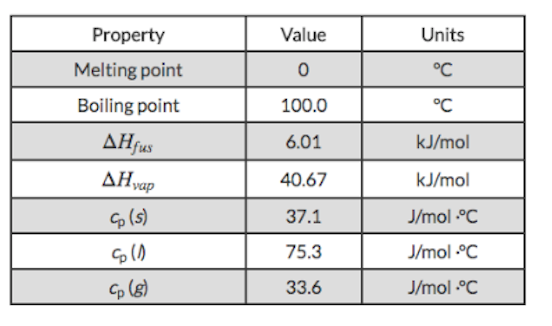# Problem: Based on the thermodynamic properties provided for water, determine the energy change when the temperature of 0.850 kg of water decreased from 123°C to 19.0°C.

###### FREE Expert Solution
79% (449 ratings)
###### FREE Expert Solution

We’re being asked to calculate the amount of energy released when 0.850 kg of water is cooled from 123 ˚C to 19.0 ˚C.

Note that cooling and freezing is an exothermic process, which means q is negative (–)

There are two heats involved in this problem:

1. q1 which is the heat in cooling 0.850 kg of H2O from 123 ˚C to 100 ˚C

2. q2 which is the heat in condensing 0.850 kg of H2O at 100 ˚C

2. q3 which is the heat in cooling 0.850 kg of H2O from 100 ˚C to 19.0 ˚C

We need to solve for each heat individually then add them together to get the final answer.

79% (449 ratings)###### Problem Details

Based on the thermodynamic properties provided for water, determine the energy change when the temperature of 0.850 kg of water decreased from 123°C to 19.0°C.Frequently Asked Questions

What scientific concept do you need to know in order to solve this problem?

Our tutors have indicated that to solve this problem you will need to apply the Heating and Cooling Curves concept. You can view video lessons to learn Heating and Cooling Curves. Or if you need more Heating and Cooling Curves practice, you can also practice Heating and Cooling Curves practice problems.

What is the difficulty of this problem?

Our tutors rated the difficulty ofBased on the thermodynamic properties provided for water, de...as medium difficulty.

How long does this problem take to solve?

Our expert Chemistry tutor, Sabrina took 12 minutes and 2 seconds to solve this problem. You can follow their steps in the video explanation above.

What professor is this problem relevant for?

Based on our data, we think this problem is relevant for Professor Cheng's class at UCR.